# Maharashtra Board 11th Maths Solutions Chapter 4 Determinants and Matrices Ex 4.5

Balbharti Maharashtra State Board Class 11 Maths Solutions Pdf Chapter 4 Determinants and Matrices Ex 4.5 Questions and Answers.

## Maharashtra State Board 11th Maths Solutions Chapter 4 Determinants and Matrices Ex 4.5

Question 1.
If A = $$\left[\begin{array}{cc} 2 & -3 \\ 5 & -4 \\ -6 & 1 \end{array}\right]$$, B = $$\left[\begin{array}{cc} -1 & 2 \\ 2 & 2 \\ 0 & 3 \end{array}\right]$$ and C = $$\left[\begin{array}{cc} 4 & 3 \\ -1 & 4 \\ -2 & 1 \end{array}\right]$$
Show that
i. A+B=B+A
ii. (A + B) + C = A + (B + C)
Solution: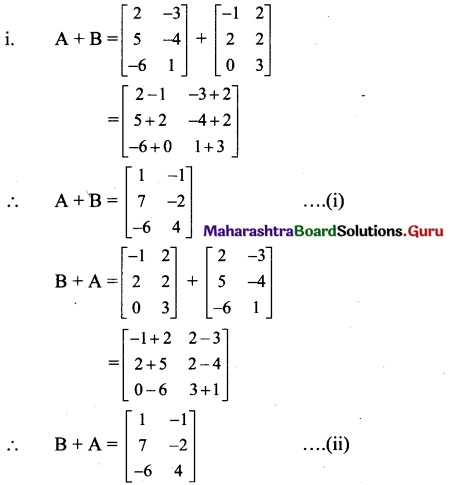From (i) and (ii), we get
A + B = B + A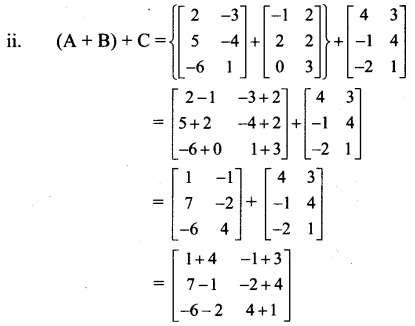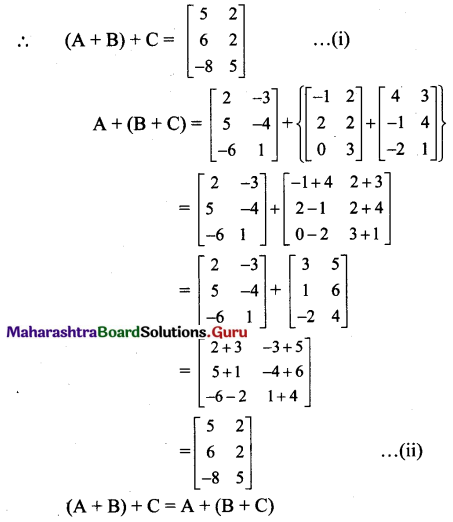Question 2.
If A = $$\left[\begin{array}{cc} 1 & -2 \\ 5 & 3 \end{array}\right]$$, B = $$\left[\begin{array}{ll} 1 & -3 \\ 4 & -7 \end{array}\right]$$ then find the matrix A – 2B + 6I, where I is the unit matrix of order 2.
Solution: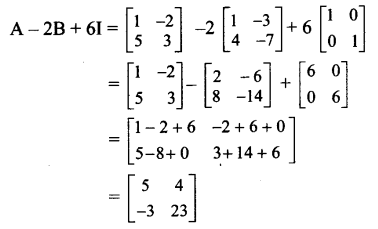Question 3.
If A = , B =  then find the matrix C such that A + B + C is a zero matrix.
Solution:
A+ B + C is a zero matrix.
∴ A + B + C = O
C = -(A + B)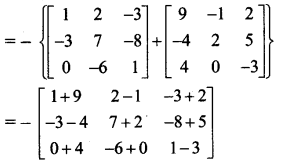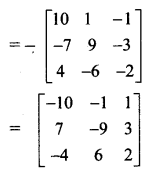Question 4.
If A = $$\left[\begin{array}{cc} 1 & -2 \\ 3 & -5 \\ -6 & 0 \end{array}\right]$$ B = $$\left[\begin{array}{cc} -1 & -2 \\ 4 & 2 \\ 1 & 5 \end{array}\right]$$ and C = $$\left[\begin{array}{cc} 2 & 4 \\ -1 & -4 \\ -3 & 6 \end{array}\right]$$ , find the matrix X such that 3A – 4B + 5X = C.
Solution:
3A-4B + 5X = C
∴ 5X = C + 4B – 3A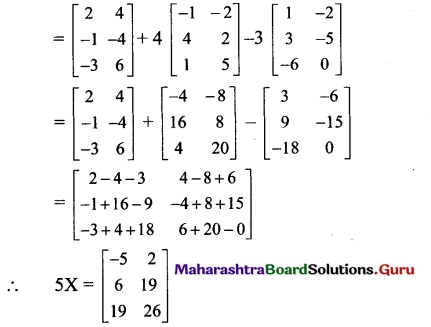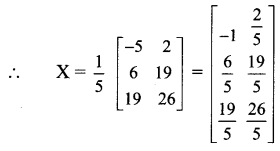Question 5.
Solve the following equations for X and Y, if 3X – Y = $$=\left[\begin{array}{cc} 1 & -1 \\ -1 & 1 \end{array}\right]$$ and X – 3Y = $$\left[\begin{array}{ll} 0 & -1 \\ 0 & -1 \end{array}\right]$$
Solution:
Given equations are
$$=\left[\begin{array}{cc} 1 & -1 \\ -1 & 1 \end{array}\right]$$……………….. (i)
and X – 3Y = $$\left[\begin{array}{ll} 0 & -1 \\ 0 & -1 \end{array}\right]$$ ………………(ii)
By (i) x 3 – (ii) we get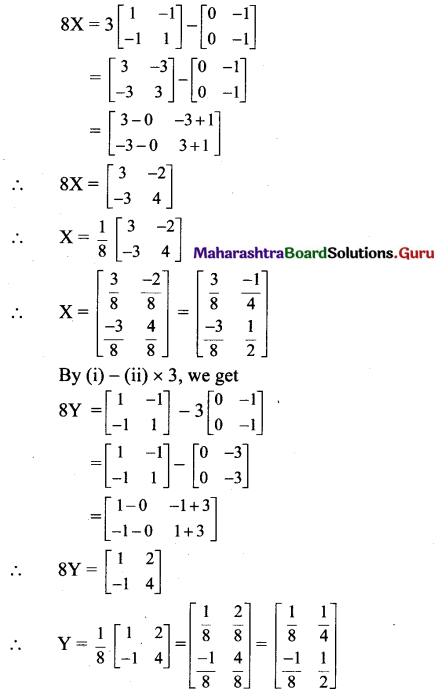Question 6.
Find the matrices A and B, if 2A – B = $$=\left[\begin{array}{ccc} 6 & -6 & 0 \\ -4 & 2 & 1 \end{array}\right]$$ and A – 2B = $$\left[\begin{array}{ccc} 3 & 2 & 8 \\ -2 & 1 & -7 \end{array}\right]$$
Solution:
Given equations are
2A – B = $$=\left[\begin{array}{ccc} 6 & -6 & 0 \\ -4 & 2 & 1 \end{array}\right]$$ ……………….. (i)
and A – 2B = $$\left[\begin{array}{ccc} 3 & 2 & 8 \\ -2 & 1 & -7 \end{array}\right]$$ ……………….(ii)
By (i) – (ii) x 2, we get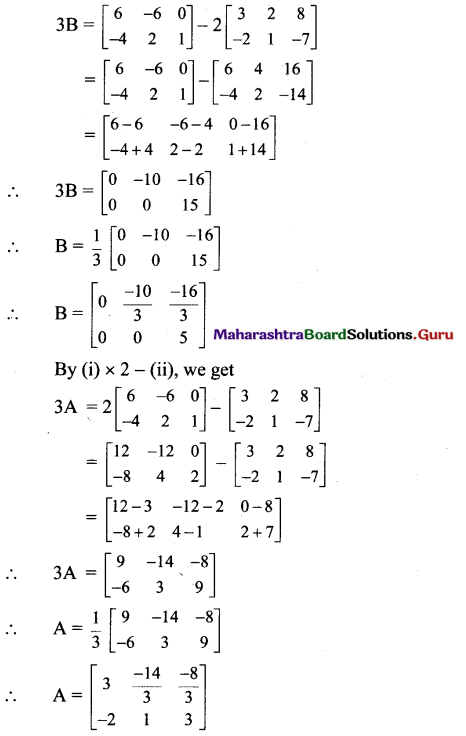Question 7.
Simplify $$\cos \theta\left[\begin{array}{cc} \cos \theta & \sin \theta \\ -\sin \theta & \cos \theta \end{array}\right]+\sin \theta\left[\begin{array}{cc} \sin \theta & -\cos \theta \\ \cos \theta & \sin \theta \end{array}\right]$$
Solution: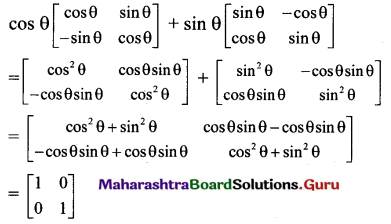Quesiton 8.
If A = $$\left[\begin{array}{cc} 1 & 2 i \\ -3 & 2 \end{array}\right]$$ and B = $$\left[\begin{array}{cc} 2 i & 1 \\ 2 & -3 \end{array}\right]$$ where i =$$\sqrt{-1}$$, find A + B and A – B. Show that A + B is singular. Is A – B singular? Justify your answer.
Solution: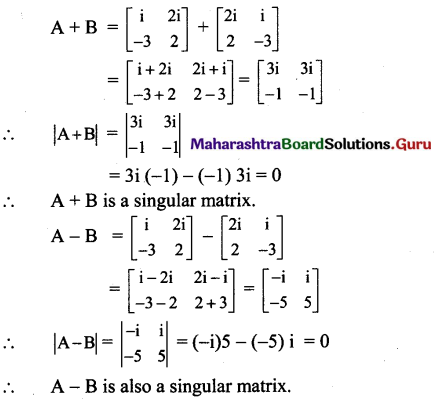Question 9.
Find x and y, if $$\left[\begin{array}{ccc} 2 x+y & -1 & 1 \\ 3 & 4 y & 4 \end{array}\right]+\left[\begin{array}{ccc} -1 & 6 & 4 \\ 3 & 0 & 3 \end{array}\right]=\left[\begin{array}{ccc} 3 & 5 & 5 \\ 6 & 18 & 7 \end{array}\right]$$
Solution: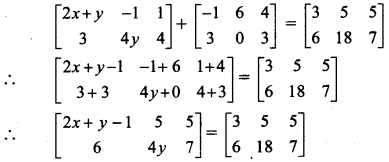∴ By equality of matrices, we get
2x + y – 1 = 3 and 4y = 18
∴ 2x + y = 4 and y = $$\frac{18}{4}=\frac{9}{2}$$
∴ 2x + $$\frac{9}{2}$$ = 4
∴ 2x = 4 – $$\frac{9}{2}$$
∴ 2x = $$\frac{1}{2}=$$
∴ x = –$$\frac{1}{4}=$$ and y = $$\frac{9}{2}=$$

Question 10.
If $$\left[\begin{array}{ll} 2 a+b & 3 a-b \\ c+2 d & 2 c-d \end{array}\right]=\left[\begin{array}{cc} 2 & 3 \\ 4 & -1 \end{array}\right]$$, find a, b, c and d.
Solution:
$$\left[\begin{array}{ll} 2 a+b & 3 a-b \\ c+2 d & 2 c-d \end{array}\right]=\left[\begin{array}{cc} 2 & 3 \\ 4 & -1 \end{array}\right]$$

∴ By equality of matrices, we get
2a + b = 2 ….(i)
3a – b = 3 ….(ii)
c + 2d = 4 ….(iii)
2c – d = -1 ….(iv)
Adding (i) and (ii), we get
5a = 5
∴ a = 1
Substituting a = 1 in (i), we get
2(1) + b = 2
∴ b = 0
By (iii) + (iv) x 2, we get
5c = 2
∴ c = $$\frac{2}{5}$$
Substituting c = $$\frac{2}{5}$$ in (iii), we get
$$\frac{2}{5}$$ + 2d = 4
∴ 2d = 4 – $$\frac{2}{5}$$
∴ 2d = $$\frac{18}{5}$$
∴ d = $$\frac{9}{5}$$
[Note: Answer given in the textbook is d = $$\frac{3}{5}$$.
However, as per our calculation it is d = $$\frac{9}{5}$$.]Question 11.
There are two book shops owned by Suresh and Ganesh. Their sales (in Rupees) for books in three subjects – Physics, Chemistry and Mathematics for two months, July and August 2017 are given by two matrices A and B.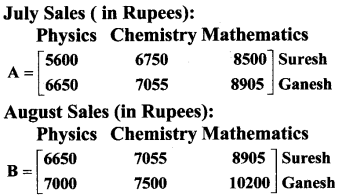i. Find the increase in sales in Rupees from July to August 2017.
ii. If both book shops got 10% profit in the month of August 2017, find the profit for each bookseller in each subject in that month.
Solution:
i. Increase in sales in rupees from July to August 2017
For Suresh:
Increase in sales for Physics books
= 6650 – 5600= ₹ 1050
Increase in sales for Chemistry books
= 7055 – 6750 = ₹ 305
Increase in sales for Mathematics books
= 8905 – 8500 = ₹ 405
For Ganesh:
Increase in sales for Physics books
= 7000 – 6650 = ₹ 350
Increase in sales for Chemistry books
= 7500 – 7055 = ₹ 445
Increase in sales for Mathematics books
= 10200 – 8905 = ₹ 1295
[Note: Answers given in the textbook are 1760, 2090. However, as per our calculation they are 1050, 305, 405, 350, 445, 1295.]

ii. Both book shops got 10% profit in the month of August 2017.
For Suresh:
Profit for Physics books = $$\frac{6650 \times 10}{100}$$ = ₹ 665
Profit for Chemistry books = $$\frac{7055 \times 10}{100}$$ = ₹ 705.50
Profit for Mathematics books = $$\frac{8905 \times 10}{100}$$ = ₹ 890.50

For Ganesh:
Profit for Physics books = $$\frac{7000 \times 10}{100}$$ = ₹ 700
Profit for Chemistry books = $$\frac{7500 \times 10}{100}$$ = ₹ 750
Profit for Mathematics books = $$\frac{10200 \times 10}{100}$$ = ₹ 1020
[Note: Answers given in the textbook for Suresh’s profit in Chemistry and Mathematics books are ? 675 and ?850 respectively. However, as per our calculation profit amounts are ₹ 705.50 and ₹ 890.50 respectively.]